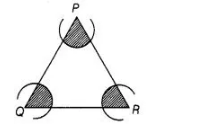# In figure, arcs have been drawn with radii 14 cm

Question:

In figure, arcs have been drawn with radii 14 cm each and with centres P, Q and R. Find the area of the shaded region.Solution:

Given that, radii of each arc (r) = 14 cm

Now, area of the sector with central $\angle P=\frac{\angle P}{360^{\circ}} \times \pi r^{2}$

$=\frac{\angle P}{360^{\circ}} \times \pi \times(14)^{2} \mathrm{~cm}^{2}$

$\left[\because\right.$ area of any sector with central angle $\theta$ and radius $\left.r=\frac{\pi r^{2}}{360^{\circ}} \times \theta\right]$

Area of the sector with central angle $=\frac{\angle Q}{360^{\circ}} \times \pi r^{2}=\frac{\angle Q}{360^{\circ}} \times \pi \times(14)^{2} \mathrm{~cm}^{2}$

and area of the sector with central.angle $R=\frac{\angle R}{360^{\circ}} \times \pi^{2}=\frac{\angle R}{360^{\circ}} \times \pi \times(14)^{2} \mathrm{~cm}^{2}$

Therefore, sum of the areas $\left(\right.$ in $\left.\mathrm{cm}^{2}\right)$ of three sectors

$=\frac{\angle P}{360^{\circ}} \times \pi \times(14)^{2}+\frac{\angle \theta}{360^{\circ}} \times \pi \times(14)^{2}+\frac{\angle R}{360^{\circ}} \times \pi \times(14)^{2}$

$=\frac{\angle P+\angle Q+\angle R}{360} \times 196 \times \pi=\frac{180^{\circ}}{360^{\circ}} \times 196 \pi \mathrm{cm}^{2}$

[since, sum of all interior angles in any triangle is $180^{\circ}$ ]

$=98 \pi \mathrm{cm}^{2}=98 \times \frac{22}{7}$

$=14 \times 22=308 \mathrm{~cm}^{2}$

Hence, the required  area of the shaded region is 308 cm2.